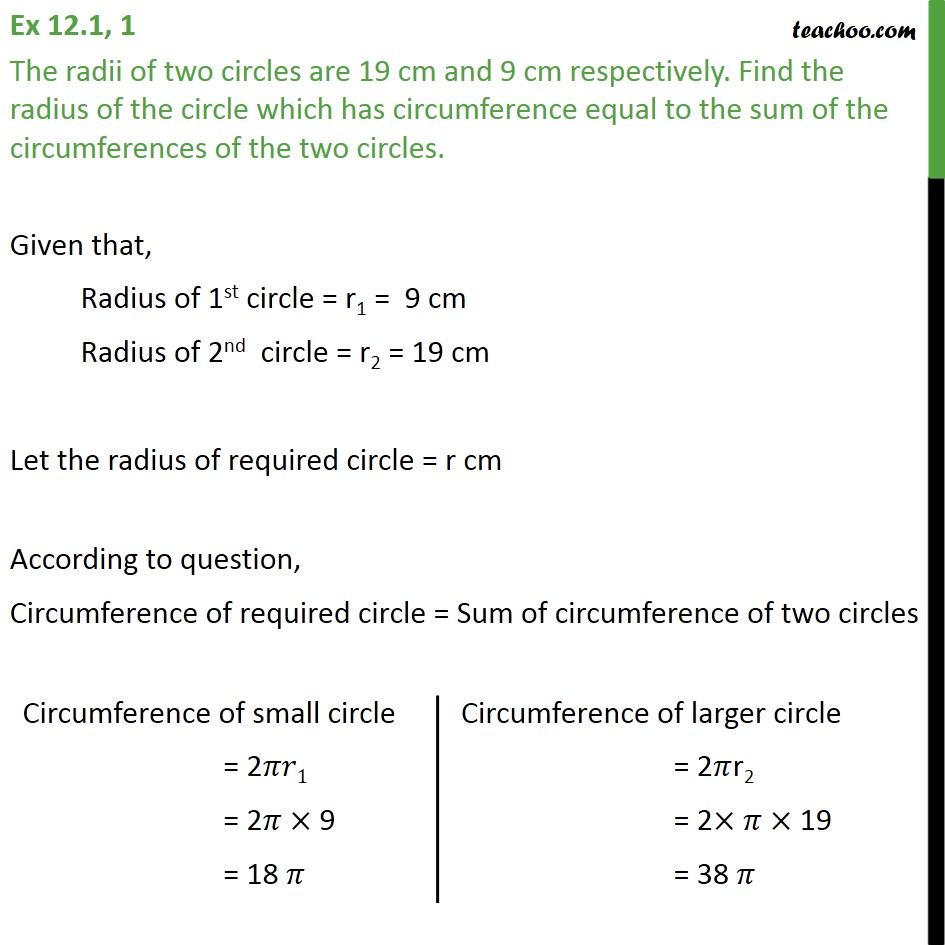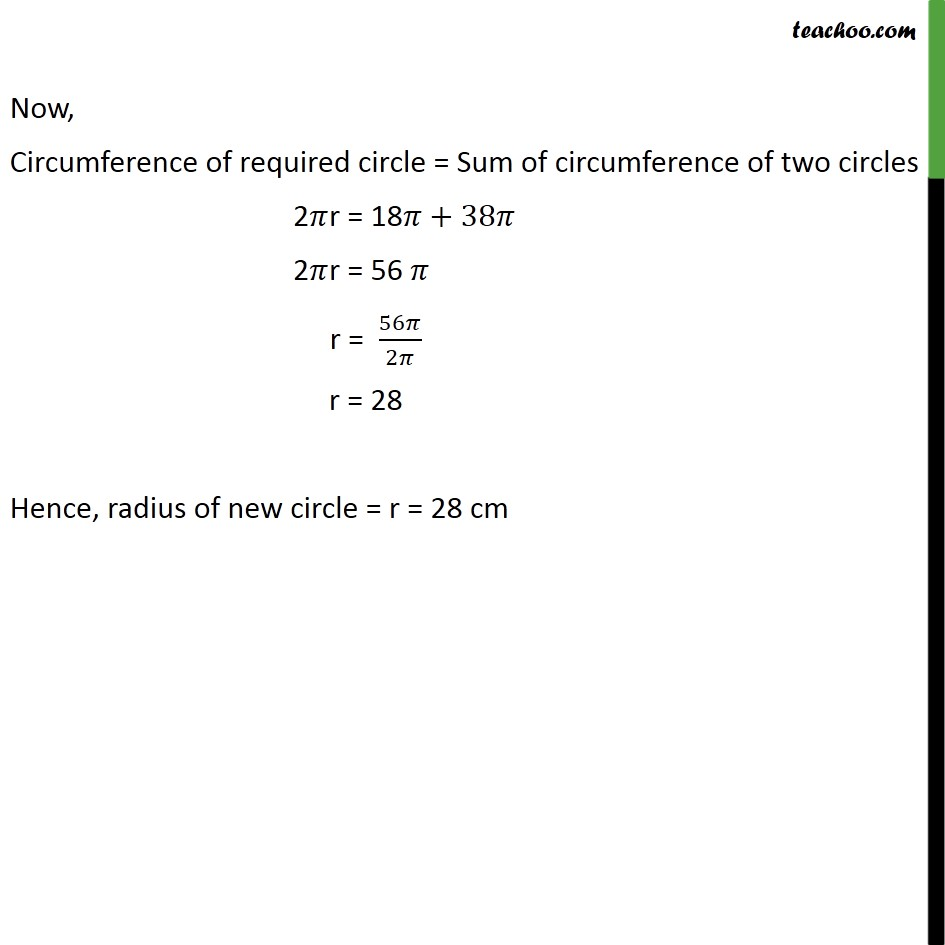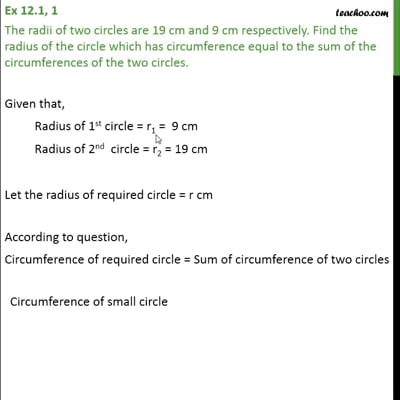Ex 12.1

Chapter 12 Class 10 Areas related to Circles (Term 1)
Serial order wiseThis video is only available for Teachoo black users

### Transcript

Ex 12.1, 1 The radii of two circles are 19 cm and 9 cm respectively. Find the radius of the circle which has circumference equal to the sum of the circumferences of the two circles. Given that, Radius of 1st circle = r1 = 9 cm Radius of 2nd circle = r2 = 19 cm Let the radius of required circle = r cm According to question, Circumference of required circle = Sum of circumference of two circles Now, Circumference of required circle = Sum of circumference of two circles 2𝜋r = 18𝜋+38𝜋 2𝜋r = 56 𝜋 r = 56𝜋/2𝜋 r = 28 Hence, radius of new circle = r = 28 cm